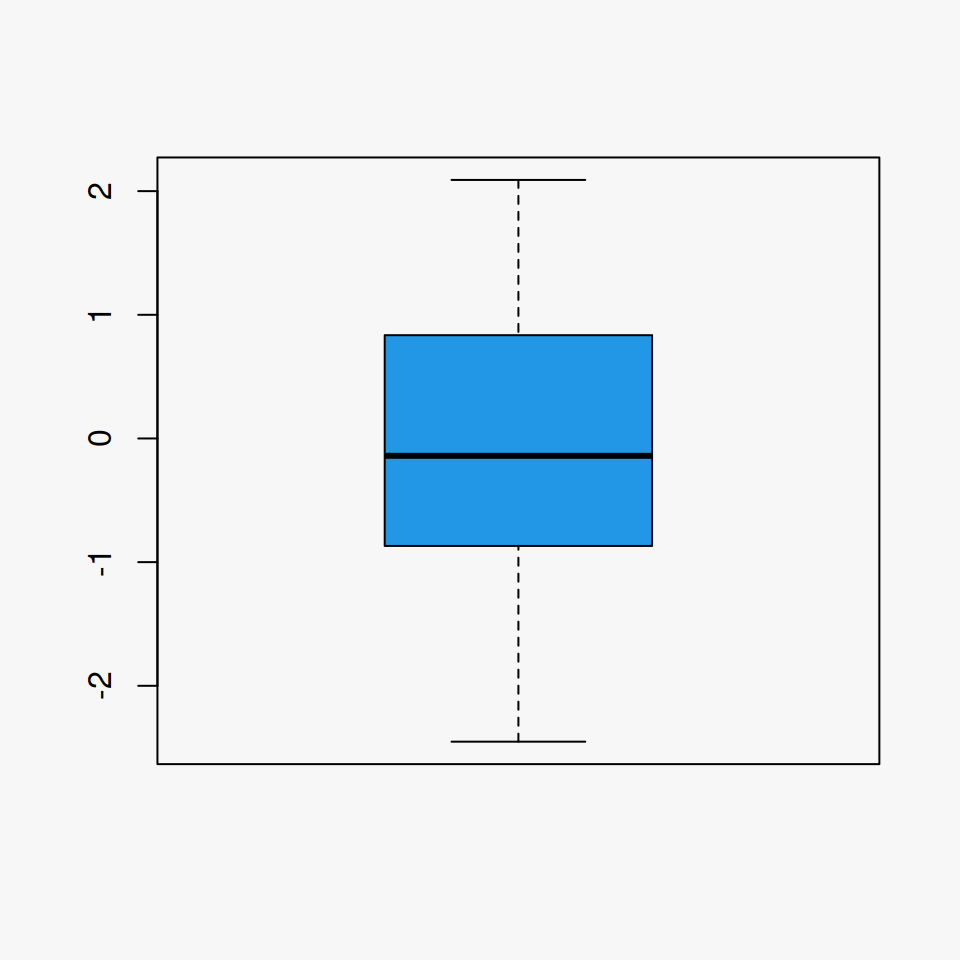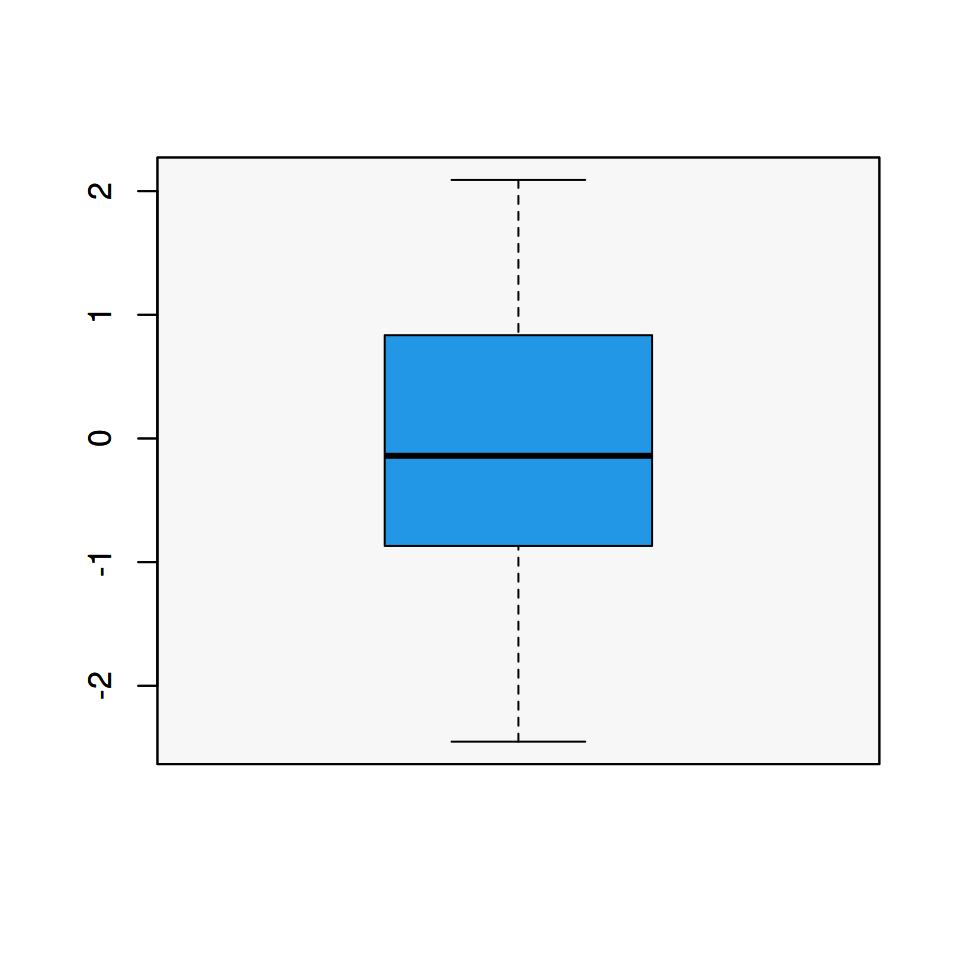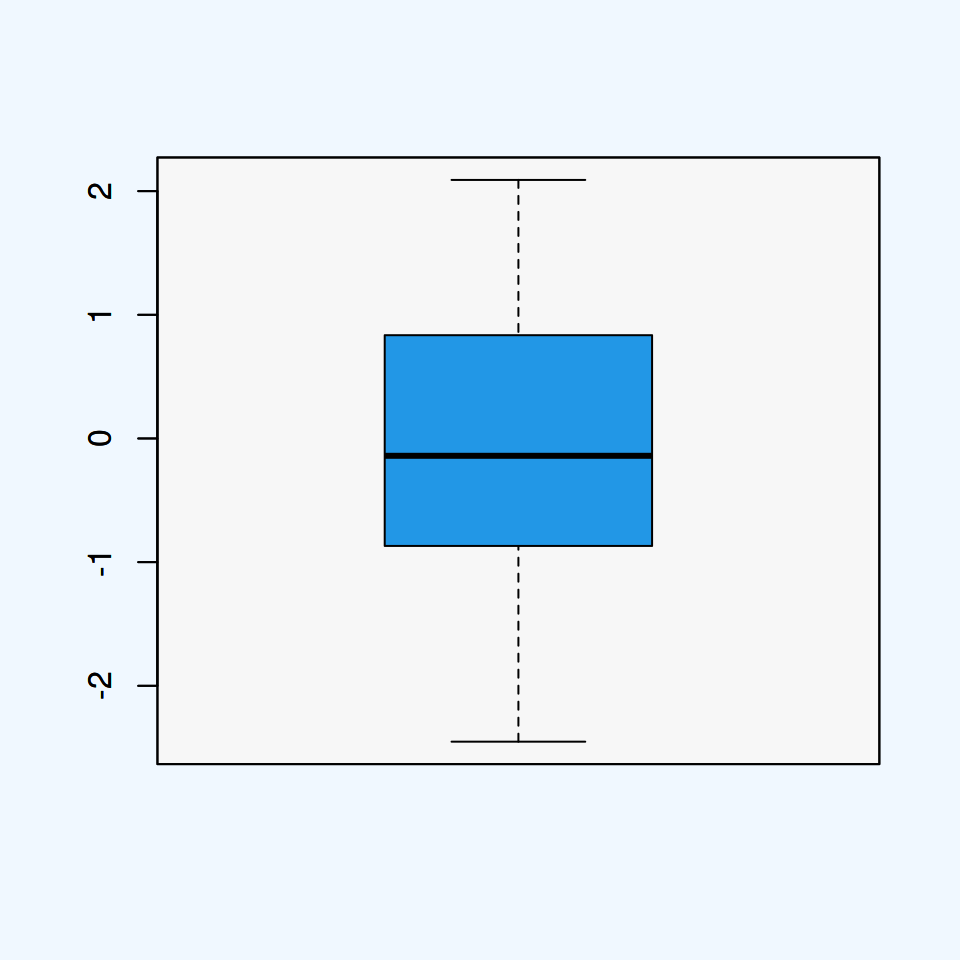# Background color in R

By default, base R charts have a white background. However, you can customize the plot background, the plot region color or both.

## Background color of the plot area

You can customize all the background color of the entire plot area with the `bg` argument of the `par` function. This will override all the background color of your plots unless you set back to the original graphical parameters.

``````# Data
set.seed(2)
x <- rnorm(100)

# Light gray background color
par(bg = "#f7f7f7")

boxplot(x, col = "blue")

# Back to the original color
par(bg = "white")``````## Background color of the plot region

A more aesthetic option is to change only the color of the plot region (also known as plot panel). This can be achieved making use of the `rect` function, specifying the coordinates of the plot region and a color.``````# Data
set.seed(2)
x <- rnorm(100)

# Create basic plot
boxplot(x)

# Plot region color
rect(par("usr"), par("usr"),
par("usr"), par("usr"),
col = "#f7f7f7") # Color

par(new = TRUE)

boxplot(x, col = 4)``````

## Plot area and plot region background color

The last option is specifying a different color for the plot and for the plot region. This can be achieved merging the approaches described above.

``````# Data
set.seed(2)
x <- rnorm(100)

# Aliceblue background color
par(bg = "aliceblue")

# Create basic plot
boxplot(x)

# Change the plot region color
rect(par("usr"), par("usr"),
par("usr"), par("usr"),
col = "#f7f7f7") # Color